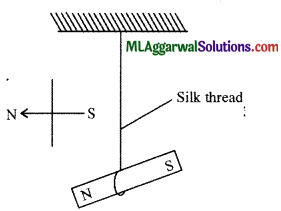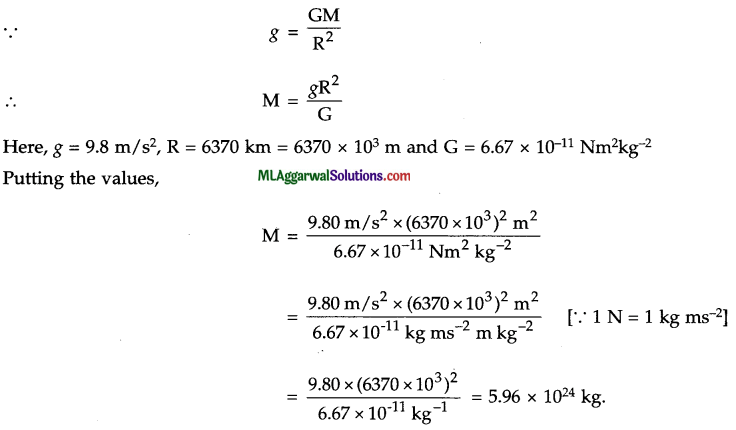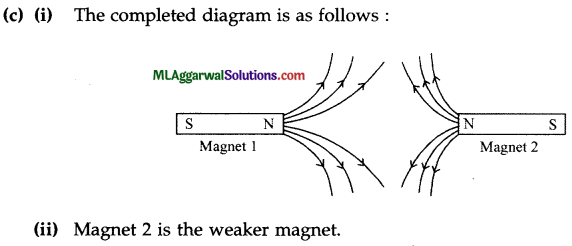## ICSE Class 9 Physics Sample Question Paper 10 with Answers

Section -1
(Attempt all questions from this section)

Question 1.
(a) The size of a particle is 5.2 p. Express it in metre.
(b) State the numerical value of the frequency of oscillation of a second’s pendulum. Does it depend on the amplitude of oscillation ?
(c) In an instrument, there are 25 divisions on the vernier scale which have length of 24 divisions of the main scale. 1 cm on main scale is divided into 20 equal parts. Find the least count.
(d) What are fundamental quantities ? Give examples.
(e) A pendulum makes 80 oscillations in 20 s. Find its frequency and time period.
(a) Given: Size of particle = 5.2 μ
Since 1μ =10-1 m
∴ Size of particle = 5.2 x 10-6 m.(b) The frequency of oscillation of a second’s pendulum is 0.5 s. No, it does not depend on the amplitude of oscillation.

(c) The value of one main scale division, x $$\frac{1}{20}$$ = cm
The number of divisions on vernier scale, n = 25
∴ Least count of vernier scale = $$=\frac{\text { Value of one main scale division }(x)}{\text { Number of divisions on vernier scale }(n)}$$
$$=\frac{\frac{1}{20}}{25}=\frac{1}{500}=0.002 \mathrm{~cm}$$

(d) Fundamental quantities are those quantities which do not depend upon other quantities. The examples are : Mass, length, time etc.

(e) Given, Oscillations in 20 s = 80
∴ Oscillations in 1 s = $$\frac{80}{20}=4$$
∴ Frequency = 4 Hz
Also $$\text { Time period }=\frac{1}{\text { Frequency }}=\frac{1}{4}=0.25 \mathrm{~s}$$

Question 2.
(a) An athlete takes 3 rounds of a circular field of radius 7 m. Find his distance and displacement.
(b) Why do dust particles from a carpet get removed when hit with a stick ?
(c) A body weighs 75 g in air, 70.5 g in water and 66 g in a liquid. Calculate the relative density of the liquid.
(f) If a stone and a pencil are dropped simultaneously in vacuum from the top of a tower, which of the two will reach the ground first ? Give reason.
(g) What can you say about the nature of motion of a body if its displacement-time graph is
(i) a straight line parallel to time axis ? (ii) a straight line inclined to the time-axis with an acute angle ?
Answer: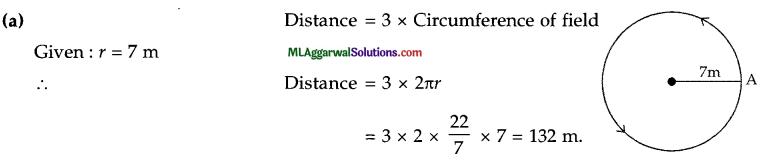Displacement = 0, because initial and final position of the athlete are the same.
(b) When a carpet is hit with a stick, it sets the carpet in motion, whereas the dust particles embedded in it remain in the initial position of rest due to inertia. Thus, the dust particles are removed from the carpet.(c) Given : Weight of body in air, W = 75.0 g
Weight of body in water, W1= 70.5 g
Weight of body in liquid, W2 = 66.0 g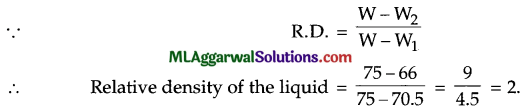(d) Both, the stone and the pencil will reach the ground simultaneously in vacuum since acceleration due to gravity is same on both.
(e) (i) The body is stationary (or no motion).
(ii) The motion is away from the starting point with uniform velocity.

Question 3.
(a) Why are we looking at alternative sources of energy ?
(b) Name the mirror which always produces an erect and virtual image. How is the size of image related to the size of the object ?
(c) At what maximum distance the image in a convex mirror can be obtained ? What will be the location of the object then ?
(d) State two laws of reflection of light.
(e) Calculate : (i) wavelength, (ii) time period of a tuning fork of frequency 480 Hz, which is set to vibrate. (Take velocity of sound in air as 332 ms-1.)
(a) We are looking at alternative sources of energy mainly due to two reasons :
(1) The fossil fuels and nuclear fuels present in the earth are limited and may not last for long, and
(2) Because of the undesirable effects of pollution both from the burning of fossil fuels and from the radioactive wastes of the nuclear power plants.

(b) A convex mirror always produces an errect and virtual image. The image formed is shorter than the object.

(c) The maximum distance at which an image in a convex mirror can be obtained is the focal length. The location of the object will be at infinity.(d) A light ray obeys the following two laws of reflection :
(1) The angle of incidence i is equal to the angle of reflection r, i.e., ∠i = ∠r.
(2) The incident ray, the reflected ray and the normal at the point of incidence, lie in the same plane.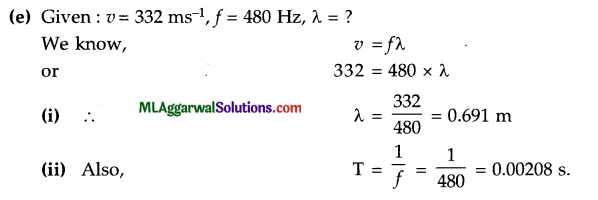Question 4.
(a) Fill in the following blanks with suitable words :
A current is a flow of………………….. for this to happen, there must be a …………..circuit
(b) Name and define the unit of current.
(c) What is meant by saying that the potential difference between two points is 1 V ?
(d) Why does a compass needle get deflected when brought near a bar magnet ?
(e) An electric bulb draws a current of 0.25 A for 10 minute. Calculate the amount of electric charge that flows through the circuit.
(a) Electrons, closed.
(b) The S.I. unit of electric current is ampere. When 1 coulomb of charge flows through any cross-section of a conductor in 1 second, the current flowing through it is said to be 1 ampere
(c) The potential difference between two points is said to be 1 volt (IV) if 1 joule of work is done in moving 1 coulomb of electric charge from one point to the other.
(d) A compass needle gets deflected when brought near a bar magnet because the bar magnet exerts a magnetic force on the compass needle which is itself a tiny pivoted magnet capable pf moving in a horizontal plane.
(e) Given : Current, I = 0.25 A, Charge, Q = ?, Time, t = 10 minute = 10 x 60 s = 600 sThus, the amount of electric charge that flows through the circuit is 150 C.

Section – II
(Attempt any four questions from this section)

Question 5.
(a) Name three main parts of a screw gauge and state their function.
(b) A particle initially at rest, moves with an acceleration 5 ms-2 for 5 s. Find the distance travelled in
(i) 4 s (ii) 5 s and (iii) 5th second.
(c) A stone is thrown upwards at 50 ms-1. Find : (i) the maximum height attained by the stone, (ii) the time taken by the stone to reach back. (Take g = – 10 ms-2.)
(a) Three parts of a screw gauge and their functions are :
(i) Sleeve : To mark main scale and base line.
(ii) Thimble : To mark circular scale.
(iii) Circular scale : Helps to read length correct upto 0.01 mm.(b) Given : Initial velocity, u = 0, Acceleration, a = 5 ms-2
(i) Distance travelled in t = 4 s,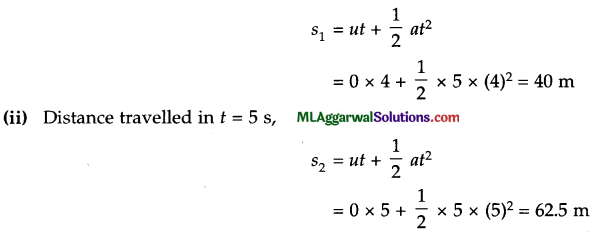(iii) Distance travelled in 5th second = Distance travelled in 5 s – Distance travelled in 4 s

(c) Given : u = 50 m/s, g = – 10 m/s2, ν = 0
(i) ν2 = u2 + 2gh
0 – (50)2 + 2 x (-10) x hQuestion 6.
(a) (i) What is ultrasound ?
(ii) State two properties of ultrasound that make it useful to us.

(b) (i) What do you mean by audible range of frequency ?
(ii) What is the audible range of frequency for a human ?
(iii) For which range of frequencies are human ears most sensitive ?

(c) How do the following factors affect, if at all, the speed of sound in air :
(i) Frequency of sound,
(ii) Temperature of air
(iii) Pressure of air
(iv) Moisture in air.
(a) (i) Sound of frequency greater than 20 kHz is known as ultrasound.
(ii) The two properties of ultrasound that make it useful to us are :
(1) High energy content, and (2) High directivity.

(b) (i) The range of frequency within which the sound can be heard by a human being is called the audible range of frequency.
(ii) The audible range of frequency for humans is 20 Hz to 20 kHz.
(iii) Human ears are most sensitive to frequencies between 2000 Hz to 3000 Hz.(c) (i) No effect
(ii) Speed of sound increases with increase in temperature.
(iii) No effect
(iv) Speed of sound increases with increase of moisture in air.

Question 7.
(a) Name the type of mirror used in the following situations :
(ii) Side/rear view mirror of a vehicle
(iii) Solar furnace.

(b) State the rules for drawing geometric images in concave mirrors.

(c) An object is placed at the following distances from a concave mirror of focal length 15 cm, turn by turn : (1) 35 cm, (2) 30 cm, (3) 20 cm, (4) 10 cm.
Which position of the object will produce :
(i) a magnified real image ?
(ii) a magnified virtual image ?
(iii) a diminished real image ?
(iv) an image of same size as the object ?
(a) (i) A concave mirror is used in the headlights of a car.
(ii) A convex mirror is used as the side/rear view mirror of a vehicle.
(iii) A concave mirror is used in a solar furnace.

(b) Rules for drawing geometric images in concave mirror are :
(1) Any ray of light travelling parallel to the principal axis of a concave mirror, after reflection passes through the principal focus of the mirror.
(2) Any ray of light which first passes through the principal focus, after reflection, will travel parallel to the principal axis of a concave mirror.
(3) A ray of light which first passes through the centre of curvature of a concave mirror after reflection will retrace its path.

(c) (i) 20 cm, (ii) 10 cm, (iv) 35 cm, (iv) 30 cm.Question 8.
(a) (i) What do you understand by thermal expansion of a substance ?
(ii) Name two substances which contract on heating.
(b) State three differences between temporary and permanent magnets.
(c) State four advantages of using biogas as fuel.
(a) (i) The expansion of a substance on heating is called the thermal expansion of that substance.
(ii) Two substances that contract on heating are : water from 0°C to 4°C, and silver iodide from 80°C to 141°C.

(b)

 Temporary Magnet Permanent Magnet They are made of soft iron. They are made from steel. They behave like a magnet only as long as there is an inducing magnet. They act as a magnet even on the removal of the inducing magnet. They have very poor magnetic retention. They have a very high magnetic retention.

(c) Four advantages of using biogas as fuel are as follows :
(1) Biogas is an excellent fuel which can be used for cooking.
(2) Biogas does not produce any smoke and hence, helps in cutting down air pollution.
(3) Biogas, on burning, leaves behind no ash.
(4) The calorific value of biogas is very high.

Question 9.
(a) (i) What is a real image ?
(ii) What type of mirror can be used to obtain a real image of an object ?
(iii) Does the mirror mentioned in part (ii) form real image for all locations of the object ?

(b) (i) At what temperature, the density of water is maximum ? State its value.
(ii) Draw a graph to show the variation in density of water with temperature in the temperature range from 0°C to 10°C.

(c) A concave lens of focal length 15 cm forms an image 10 cm from the lens. How far is the object from the lens ?
(a) (i) A real image is one which can be obtained on a screen.
(ii) A concave mirror can be used to obtain a real image of an object.
(iii) No, it forms a virtual image if the object is placed between the focus and pole of the mirror.(b) (i) The density of water is maximum at a temperature of 4°C and its value is 1000 kg m3.
(ii) The graph showing variation in density of water with temperature in range 0°C to 10°C is shown below.(c) Given : Object distance, u = ?
Image distance, ν = – 10 cm
Focal length, f= – 15 cm
Putting these values in the lens formula :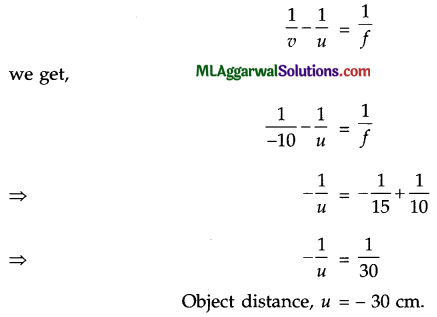Thus, the object is placed at a distance of 30 cm from the concave lens. The minus sign with the object distance shows that the object is on its left side, i.e., in front of mirror.

Question 10.
(a) A small magnet is suspended by a silk thread from a rigid support such that the magnet can freely swing. How will it rest ? Draw a diagram to show it.
(b) Calculate the mass of the earth, assuming it to be a sphere of radius 6370 km. (Take G = 6.67 x 10-11 Nm2 kg-2 and g = 9.80 ms-2.)
(c) The given figure shows the magnetic field between two magnets.